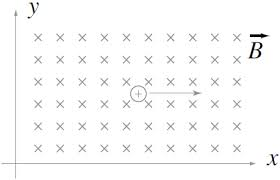# Foolish $\alpha$ Particle in Variable Magnetic FieldLet a variable magnetic field exist in $XYZ$ plane only in 1st quadrant, such that its magnitude is varying with distance $x$ $(B={ B }_{ 0 }x)$ and direction in positive $Z$-axis.

Now an $\alpha$-particle of mass $m$ and charge $q$ enters in this magnetic field at origin with velocity ${V}_{0}$ and direction in positive $X$-axis.

Then find the radius of curvature of trajectory of particle at the instant when the particle displaced maximum distance in $X$-direction

Details and assumptions

1)${ B }_{ 0 }=1.67\times { 10 }^{ -27 }T$

2)${ V }_{ 0 }=1.28\times { 10 }^{ -18 }m/s$

3)${ m }_{ \alpha }=6.68\times { 10 }^{ -27 }Kg$

4)${ q }_{ \alpha }=3.2\times { 10 }^{ -19 } C$

×